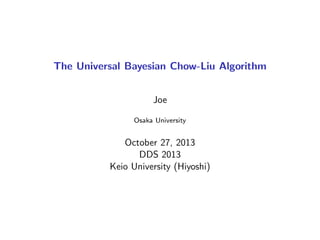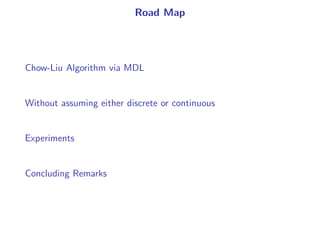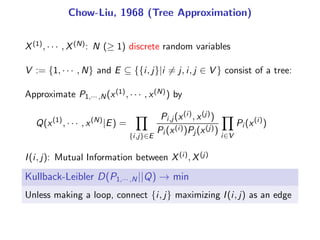Successfully reported this slideshow.

# The Universal Bayesian Chow-Liu Algorithm

0

Share×
1 of 17
1 of 17

# The Universal Bayesian Chow-Liu Algorithm

0

Share

The Universal Bayesian Chow-Liu Algorithm, DDS, Oct. 2013

The Universal Bayesian Chow-Liu Algorithm, DDS, Oct. 2013

## More Related Content

### Related Books

Free with a 14 day trial from Scribd

See all

### Related Audiobooks

Free with a 14 day trial from Scribd

See all

### The Universal Bayesian Chow-Liu Algorithm

1. 1. The Universal Bayesian Chow-Liu Algorithm Joe Osaka University October 27, 2013 DDS 2013 Keio University (Hiyoshi)
2. 2. Road Map Chow-Liu Algorithm via MDL Without assuming either discrete or continuous Experiments Concluding Remarks
3. 3. Chow-Liu, 1968 (Tree Approximation) X(1), · · · , X(N): N (≥ 1) discrete random variables   V := {1, · · · , N} and E ⊆ {{i, j}|i = j, i, j ∈ V } consist of a tree:   Approximate P1,··· ,N(x(1), · · · , x(N)) by Q(x(1) , · · · , x(N) |E) = {i,j}∈E Pi,j (x(i), x(j)) Pi (x(i))Pj (x(j)) i∈V Pi (x(i) ) I(i, j): Mutual Information between X(i), X(j) Kullback-Leibler D(P1,··· ,N||Q) → min Unless making a loop, connect {i, j} maximizing I(i, j) as an edge
4. 4. Example i 1 1 2 1 2 3 j 2 3 3 4 4 4 I(i, j) 12 10 8 6 4 2 ❥ ❥ ❥ ❥ 2 4 1 3 ❥ ❥ ❥ ❥ 2 4 1 3 ❥ ❥ ❥ ❥ 2 4 1 3 ❥ ❥ ❥ ❥ 2 4 1 3 ❅❅
5. 5. Why Chow-Liu works? D(P1,··· ,N||Q) = −H(1, · · · , N) + N i∈V H(i) − {i,j}∈E I(i, j)   Kruskal’s Algorithm: ◮ Unless making a loop, connects e ∈ E maximizing w(e) ◮ constructs a tree (V , E) maximizing e∈E w(e) w : E → R≥0
6. 6. Chow-Liu: Tree Learning via Maximum Likelihood Learning rather than Approximation Starting from n examples xn = {(x (j) i )N j=1}n i=1 rather than P1,··· ,N Calculate relative frequencies ˆpi , ˆpi,j given xn to obtain   ˆHn (xn |E) := n i∈V ˆH(i) − n {i,j}∈E ˆI(i, j) Empirical entropy ˆHn (xn |E) → min Unless making a loop, connect {i, j} maximizing ˆI(i, j) as an edge
7. 7. Chow-Liu: Tree Learning via MDL (Suzuki, 1993) π(E): Prior Probability of E assuming to be uniform Description Length of xn under E: L(xn |E) := ˆHn (xn |E) + 1 2 k(E) log n # of Parameters: k(E) := i∈V α(i) + {i,j}∈E (α(i) − 1)(α(j) − 1) α(i): the # of values X(i) takes Description Length L(xn |E) − log π(E) → min Unless making a loop, connect {i, j} maximizing ˆJ(i, j) as an edge ˆJ(i, j) = ˆI(i, j) − 1 2n (α(i) − 1)(α(j) − 1) log n
8. 8. ML vs MDL ML MDL Choice ˆHn(xn|E) ˆHn(xn|E) + 1 2k(E) log n of E → min → max Choice ˆI(i, j) ˆI(i, j) of {i, j} → max − 1 2n (α(i) − 1)(α(j) − 1) log n → max Criteria Fitness of xn to E Fitness of xn to E Simplicity of E Target Trees Forests Correctness Overestimation Correct as n grows ML seeks a tree even if the random variables are independent.
9. 9. Chow-Liu: Tree Learning via Bayes (Suzuki, 2012) Replace {Pi (x(i))}i∈V and {Pi,j (x(i), x(j))}{i,j}∈E in Q1,··· ,N(x(1) , · · · , x(N) |E) = {i,j}∈E Pi,j(x(i), x(j)) Pi (x(i))Pj (x(j)) i∈V Pi (x(i) ) by {R(i)}i∈V and {R(i, j)}{i,j}∈E in Rn (xn |E) := {i,j}∈E Rn(i, j) Rn(i)Rn(j) i∈V Rn (i) Posterior Probability π(E)Rn (xn |E) → max Unless making a loop, connect {i, j} maximizing J(i, j) as an edge J(i, j) := 1 n log Rn(i, j) Rn(i)Rn(j)
10. 10. MDL reduces to Bayes If we choose {R(i)} and {R(i, j)} properly, L(xn |E) = − log Rn (xn |E) ⇐⇒ Rn (xn |E) = 2−L(xn|E) ˆJ(i, j) = J(i, j) Universality: require Rn (·|·) to satisfy 1 n log Qn(xn|E) Rn(xn|E) → 0 as n → ∞ for any Qn(·|·).
11. 11. zip, gzip, uuencode, lzh, etc A: ﬁnite set ϕ : An → {0, 1}∗ (Data Compression) l(xn): the output length of xn ∈ An w.r.t. ϕ   Rn(xn) := 2−l(xn) satisﬁes 1 n log Pn(xn) Rn(xn) → 0 as n → ∞ for any Probability Pn(xn) of xn ∈ An
12. 12. Without assuming {X(i) }N i=1 to be discrete In any database some ﬁelds are discrete others continuos: ◮ discrete (ﬁnite/inﬁnite) ◮ continuous ◮ others (neither discrete or continuous) Neither discrete or continuous (Suzuki, 2011) constructed generalized universal density function gn(xn) 1 n log f n(xn) gn(xn) → 0 as n → ∞ for any generalized probability density function f n(xn)
13. 13. Example {Aj }: Aj+1 is a reﬁnement of Aj . A1 = {[0, 1/2), [1/2, 1)} A2 = {[0, 1/4), [1/4, 1/2), [1/2, 3/4), [3/4, 1)} . . . Aj = {[0, 2−(j−1)), [2−(j−1), 2 · 2−(j−1)), · · · , [(2j−1 − 1)2−(j−1), 1)} . . . sj : A → Aj (quantization, x ∈ a ∈ Aj =⇒ sj (x) = a) λ: Lebesgue measure (width) Qn j : universal measure w.r.t. Aj (sj (x1), · · · , sj (xn)) = (a1, · · · , an) =⇒ gn j (xn ) := Qn j (a1, · · · , an) λ(a1) · · · λ(an) {ωj }: ωj > 0, j ωj = 1 gn (xn ) = j ωjgn j (xn )
14. 14. Bayesian Estimator of Mutual Information J(i, j) := 1 n log Rn(i, j) Rn(i)Rn(j) X(i), X(j) may be any pair of discrete, or continuous, or others   Previous Work Liang, 2004 only deals with discrete variables (after Suzuki, 1993) Edwords, et.al 2010 assumes that the discrete and continuous variables are connected.
15. 15. Experiments using R juul2 data frame in the ISwR package contains insulin-like growth factor, one observation per subject in various ages, with the bulk of the data collected in conection with physical examinations. n = 1336 setClass("random.variable", representation(data="numeric", breaks="array",width="array")) setClass("continuous", contains="random.variable", representation(mu="numeric",sigma="numeric")) setClass("discrete", contains="random.variable") X[]<-new("continuous", data=juul2\$age) X[]<-new("continuous", data=juul2\$height) X[]<-new("discrete", data=juul2\$menarche) X[]<-new("discrete", data=juul2\$sex) X[]<-new("discrete", data=juul2\$igf1) X[]<-new("discrete", data=juul2\$tanner) X[]<-new("discrete", data=juul2\$testvol) X[]<-new("continuous", data=juul2\$weight)
16. 16. Actual Computation of J(i, j) age height menarche sex igf1 tanner testvol we age * height 1.6923 * menarche 2.9892 1.0102 * sex 2.6212 1.9865 1.2209 * igf1 0.9821 1.8790 1.1872 1.2982 * tanner 0.8781 0.7652 0.8765 1.1187 0.9827 * testvol 0.8782 0.9876 1.2987 1.9981 0.6651 0.7612 * weight 0.9872 1.7121 3.9812 0.9123 0.4581 1.0921 1.2201
17. 17. Concluding Remarks n: sample size N: # of variables L: the maximum depth of quantizations Computation O(n), O(N2), and O(L) Bayesian Chow-Liu Algorithm In any database, some ﬁelds are discrete and others are continuous. ◮ Easy programming ◮ Fast Computation Future Work: constructing a R command Currently, specify either discrete or continuous for each variable.